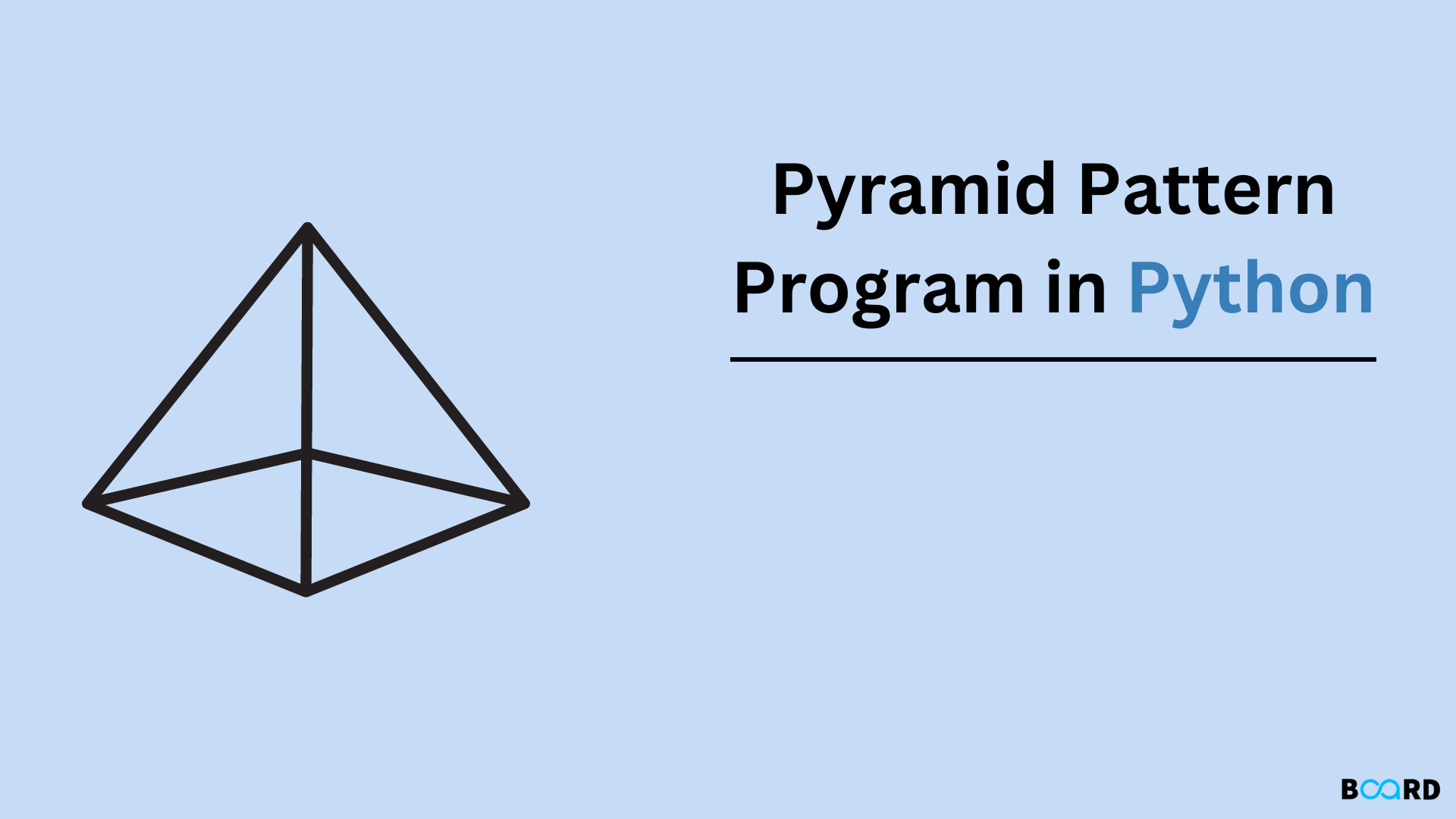# Python Pyramid Pattern Program## Introduction

Patterns can be printed in python using simple for loops. The first outer loop is used to handle the number of rows and the inner nested loop is used to handle the number of columns. Manipulating the print statements, different numbers patterns, alphabet patterns, or star patterns can be printed.

Some of the Patterns are:

## Simple Half Pyramid Pattern

Example Code:

 print("Enter no. of rows: ")number=int(input())for i in range(0,number):    for j in range(0, i+1):        print("\$",end=" ")        print()

Output:

 Enter no. of rows:6\$ \$ \$ \$ \$ \$ \$ \$ \$ \$ \$ \$ \$ \$ \$ \$ \$ \$ \$ \$ \$

Explanation:

• In the above program, we are using nested for loop, and the required variables should be declared and initialized.
• We get the value from the user to print the number of rows.
• The first ‘for’ loop starts from i=0 to i=number.
• The inner for loop starts from j=0 to j=i+1.
• The given symbol is printed for the given number of rows.

## Simple Full Pyramid Pattern

Example code:

 print("Enter no. of rows: ")rows=int(input())k=0for i in range(1,rows+1):    for j in range(1, (rows-i)+1):        print(end=" ")            while k!=(2*i-1):        print("\$",end="")        k += 1        k=0        print()

Output:

 Enter no. of rows: 7      \$     \$\$\$    \$\$\$\$\$   \$\$\$\$\$\$\$  \$\$\$\$\$\$\$\$\$ \$\$\$\$\$\$\$\$\$\$\$\$\$\$\$\$\$\$\$\$\$\$\$\$

## Approach 2: Using List in Python 3:

Example Code:

 def pattern(n):    List=[]    for i in range(1,n+1):        List.append("\$"*i)    print("\n".join(List))n=6pattern(n)

Output:

 \$\$\$\$\$\$\$\$\$\$\$\$\$\$\$\$\$\$\$\$\$

## Approach 3: Using recursion

Example code:

 def pattern(n):    if n==0:        return    else:        pattern(n-1)        print("\$ "*n)       n=4pattern(n)

Output:

 \$ \$ \$ \$ \$ \$ \$ \$ \$ \$

## Approach 4: Using a while loop

Example Code:

 n=int(input("Enter no. of rows: "))i=1;j=0while(i<=n):    while(j<=i-1):        print("\$ ",end="")        j+=1            print("\n")    j=0;i+=1

Output:

 Enter no. of rows: 9\$ \$ \$ \$ \$ \$ \$ \$ \$ \$ \$ \$ \$ \$ \$ \$ \$ \$ \$ \$ \$ \$ \$ \$ \$ \$ \$ \$ \$ \$ \$ \$ \$ \$ \$ \$ \$ \$ \$ \$ \$ \$ \$ \$ \$

## Approach 5:Using a for loop:

### Number Pattern

Example code:

 def pattern(n):    number=1    for i in range(0,n):        number = 1        for j in range(0, i+1):            print(number,end=" ")            number += 1        print("\n")n=6pattern(n)

Output:

 1 1 2 1 2 3 1 2 3 4 1 2 3 4 5 1 2 3 4 5 6

### Numbers without reassigning:

Example code:

 def pattern(n):    number=1    for i in range(0,n):        # not reassigning number=1        for j in range(0, i+1):            print(number,end=" ")            number += 1        print("\n")n=6pattern(n)

Output:

 1 2 3 4 5 6 7 8 9 10 11 12 13 14 15 16 17 18 19 20 21

### Character Pattern

Example code:

 def pattern(n):    # initializing ASCII value corresponding to 'A'    number=65    for i in range(0,n):        for j in range(0, i+1):            ch = chr(number)            print(ch,end=" ")        number += 1        print("\n")n=5pattern(n)

Output:

 A B B C C C D D D D E E E E E

### Continuous Character Pattern

Example code:

 def pattern(n):    # initializing ASCII value corresponding to 'A'    number=65    for i in range(0,n):        for j in range(0, i+1):            ch = chr(number)            print(ch,end=" ")            number += 1        print("\n")n=5pattern(n)

Output:

 A B C D E F G H I J K L M N O

## Conclusion

Once we get the logic of the program, we can print any pattern using the Python loops.